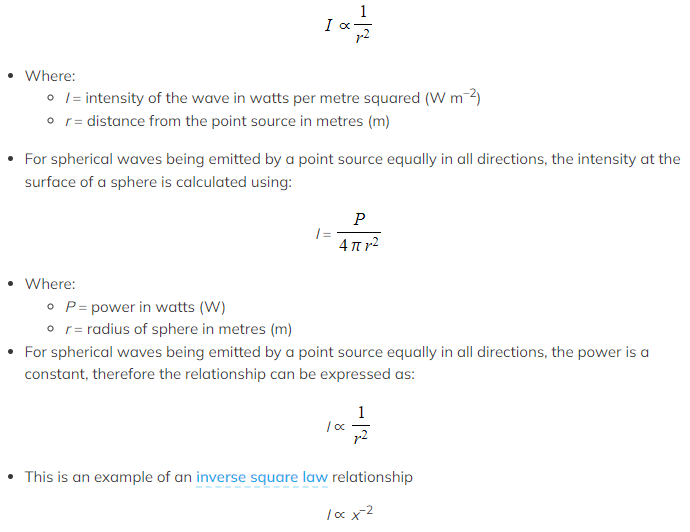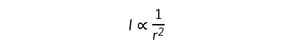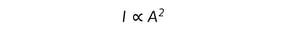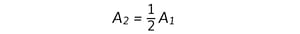# IB DP Physics: SL复习笔记4.3.2 Amplitude & Intensity

### Amplitude & Intensity

#### Intensity

• The intensity of a wave is defined as follows:

Power per unit area

• Intensity is measured in W m–2
• Power is defined as:

The rate of energy transfer

• Therefore, intensity can also be defined as:

The rate of energy transfer per unit area

• For spherical waves being emitted by a point source equally in all directions, the intensity follows an inverse square law with distance from the point sourceIntensity decreases by the inverse square law

#### Intensity Variation with Amplitude

• By definition, the intensity of a wave (its power per unit area) is proportional to the energy transferred by the wave
• The intensity of a wave at a particular point is related to the amplitude of the wave at that point
• The energy transferred by a wave is proportional to the square of the amplitude
• Therefore, the intensity of a wave is proportional to the square of the amplitude
•Where:
• I = intensity of the wave in W m–2
• A = amplitude of the wave in metres (m)

#### Worked Example

A person stands 10 m away from a loudspeaker. The sound produced by the loudspeaker is very loud, so the person moves 20 m away from it.

State the effect of this change on the intensity and the amplitude of the sound waves heard by the person.

Step 1: Write down the known quantities

• Original distance, r1 = 10 m
• New distance, r2 = 20 m

Step 2: Write down the relationship between the intensity of a wave and the distance from the point source producing the waveStep 2: State the new intensity

• Since the distance doubles (r2 = 2r1), the intensity is reduced by a factor fourStep 3: Write down the relationship between the intensity of a wave and its amplitudeStep 4: State the new amplitude

• Since the intensity is reduced by a factor four, the amplitude decreases by half